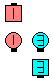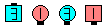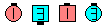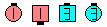Definitions of Square Dance Calls and Concepts
"Z" Axle [C3B]

Index -->  Plus  |  A1  |  A2  |  C1  |  C2  |  C3A  |  C3B  |  C4  |  NOL  |
Definitions (Text Only) -->  Plus  |  A1  |  A2  |  C1  |  C2  |  C3A  |  C3B  |  C4  |  NOL  |
 Find call:

"Z" Axle -- [C3B]
(Dave Hodson 1980)
 C3B:\$B8@8l(BFrom the "Z" obtained from a Line with the Centers in a Mini-Wave after a Step & Slide. EN: 10
Centers \$B\$,(B Mini-Wave \$B\$H\$J\$C\$F\$\$\$k(B Line \$B\$+\$i(B Step & Slide \$B\$r\$7\$FF@\$i\$l\$k(B "Z" \$B\$+\$i(B. JP: 10

1. Outsides Cross Cast Back;

Ends in a Line. "Z"-Axle is a 3-part call. EN: 30
Line \$B\$G=*\$o\$j\$^\$9(B. "Z"-Axle \$B\$O(B 3 \$B%Q!<%H\$N%3!<%k\$G\$9(B. JP: 30"Z"-Axle\$B\$NA0(B Outsides Cross Cast Back(1/3)\$B\$N8e(B all Trade(2/3)\$B\$N8e(B Centers Trade\$B\$N8e(B (\$B=*\$o\$j(B)

Pass "Z" Axle [C3B] (Dave Hodson 1980):
From the "Z" obtained from a Two-Faced Line after the Ends Fold. Pass Thru; Outsides Cross Cast Back; all Trade; Centers Trade. Ends in a Wave. This is a 4-part call. EN: 619
Two-Faced Line \$B\$+\$i(B Ends Fold \$B\$r\$7\$FF@\$i\$l\$k(B "Z" \$B\$+\$i(B. Pass Thru; Outsides Cross Cast Back; all Trade; Centers Trade. Wave \$B\$G=*\$o\$j\$^\$9(B. 4 \$B%Q!<%H\$N%3!<%k\$G\$9(B. JP: 619Choreography for "Z" AxleComments? Questions? Suggestions?

 C3B: